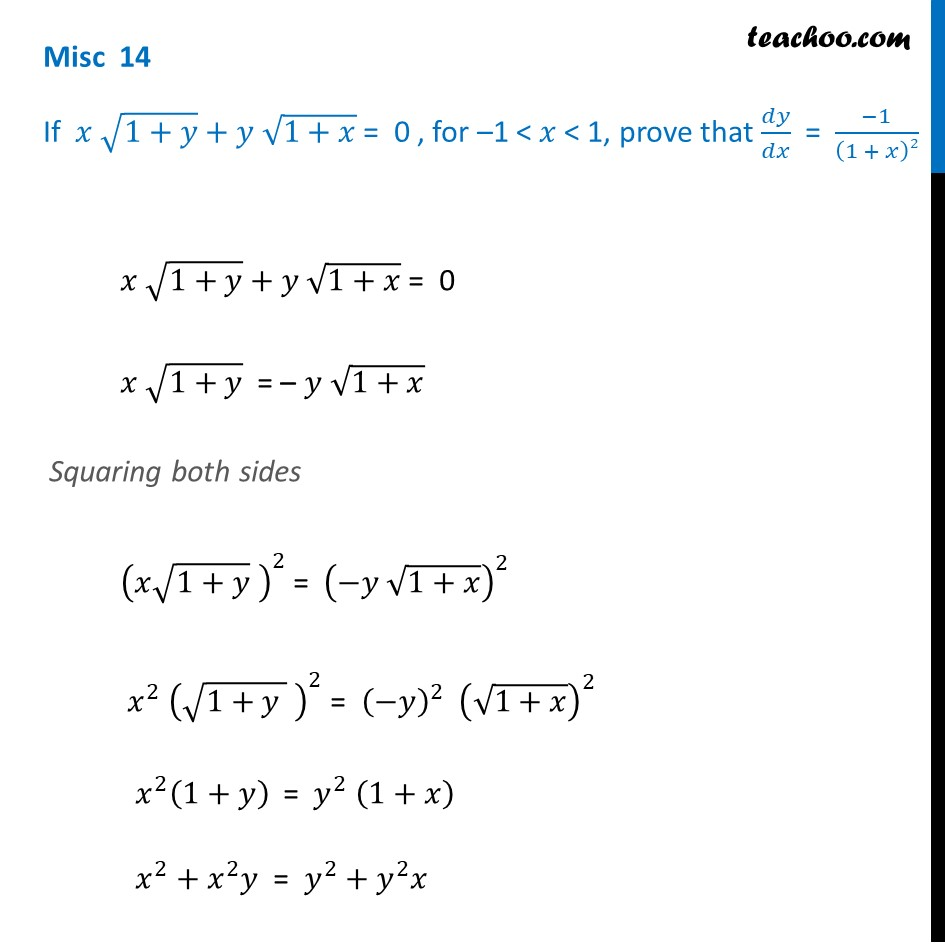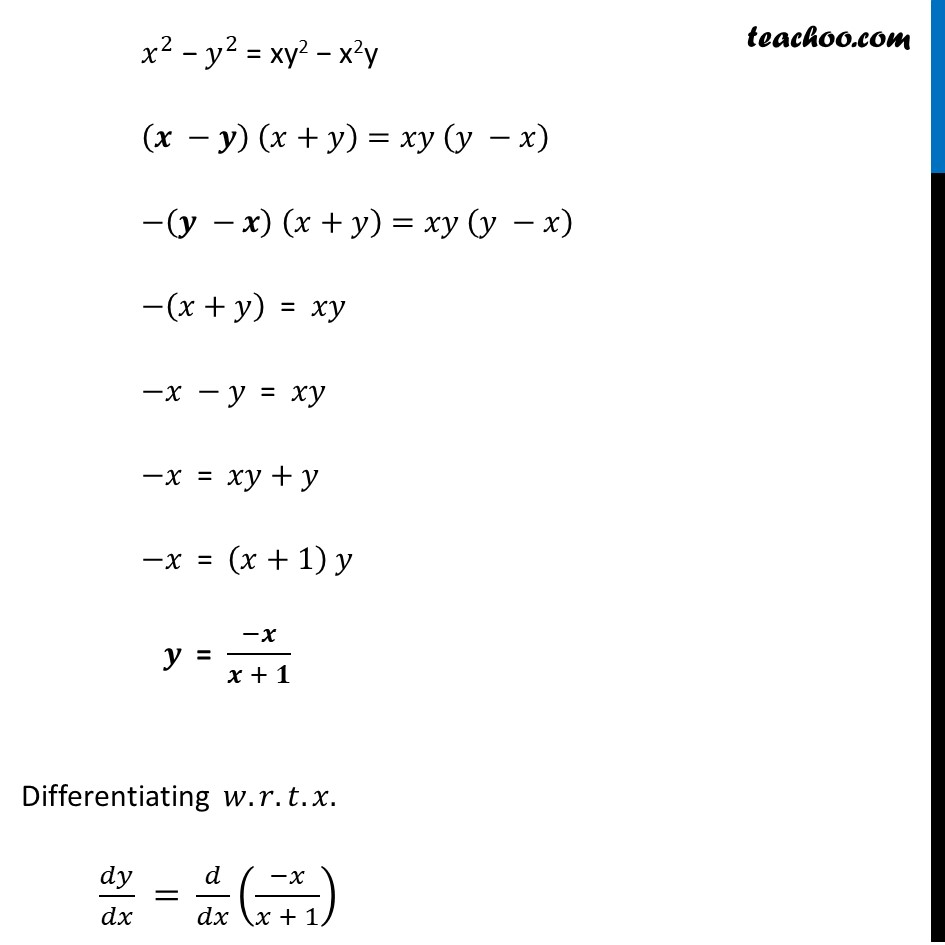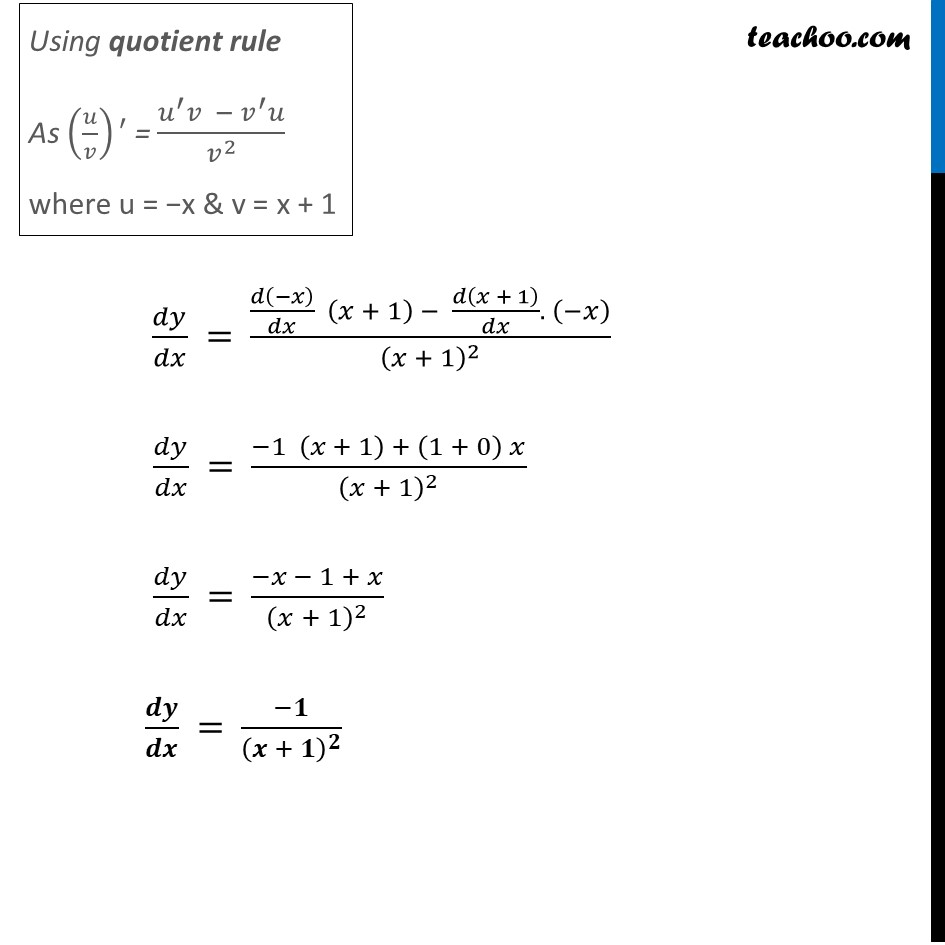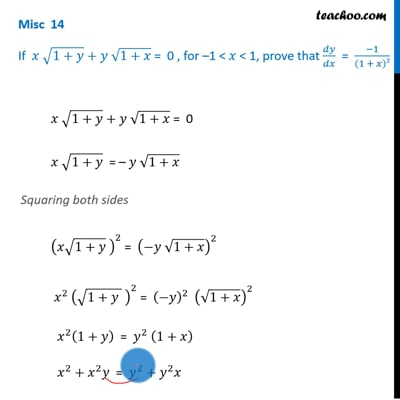Finding derivative of Implicit functions

Chapter 5 Class 12 Continuity and Differentiability
Concept wiseThis video is only available for Teachoo black users

Introducing your new favourite teacher - Teachoo Black, at only ₹83 per month

### Transcript

Misc 14 If 𝑥 √(1+𝑦)+𝑦 √(1+𝑥) = 0 , for –1 < 𝑥 < 1, prove that 𝑑𝑦/𝑑𝑥 = (−1)/(1 + 𝑥)2 𝑥 √(1+𝑦)+𝑦 √(1+𝑥) = 0 𝑥 √(1+𝑦) = – 𝑦 √(1+𝑥) Squaring both sides (𝑥√(1+𝑦) )^2 = (−𝑦 √(1+𝑥))^2 𝑥^2 (√(1+𝑦 ) )^2 = (−𝑦)^2 (√(1+𝑥))^2 𝑥^2 (1+𝑦) = 𝑦^2 (1+𝑥) 𝑥^2+𝑥^2 𝑦 = 𝑦^2 + 𝑦^2 𝑥 𝑥^2 − 𝑦^2 = xy2 − x2y (𝒙 −𝒚) (𝑥+𝑦)=𝑥𝑦 (𝑦 −𝑥) −(𝒚 −𝒙) (𝑥+𝑦)=𝑥𝑦 (𝑦 −𝑥) −(𝑥+𝑦) = 𝑥𝑦 −𝑥 −𝑦 = 𝑥𝑦 −𝑥 = 𝑥𝑦+𝑦 −𝑥 = (𝑥+1) 𝑦 𝒚 = (−𝒙)/(𝒙 + 𝟏) Differentiating 𝑤.𝑟.𝑡.𝑥. 𝑑𝑦/𝑑𝑥 = 𝑑/𝑑𝑥 ((−𝑥)/(𝑥 + 1)) Using quotient rule As (𝑢/𝑣)′ = (𝑢^′ 𝑣 − 𝑣^′ 𝑢)/𝑣^2 where u = −x & v = x + 1 𝑑𝑦/𝑑𝑥 = (𝑑(−𝑥)/𝑑𝑥 (𝑥 + 1) − 𝑑(𝑥 + 1)/𝑑𝑥. (−𝑥))/(𝑥 + 1)^2 𝑑𝑦/𝑑𝑥 = (−1 (𝑥 + 1) + (1 + 0) 𝑥)/(𝑥 + 1)^2 𝑑𝑦/𝑑𝑥 = (−𝑥 − 1 + 𝑥)/(𝑥 + 1)^2 𝒅𝒚/𝒅𝒙 = (−𝟏)/(𝒙 + 𝟏)^𝟐